﻿ Ab Initio Calculations of Electronic Properties of Si<SUB>x</SUB>Sn<SUB>1-</SUB><SUB>x</SUB> Using Becke-Johnson Modified Method

### Ab Initio Calculations of Electronic Properties of SixSn1-x Using B...

N. Amrane, M. Benkraouda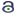OPEN ACCESS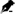PEER-REVIEWED

## Ab Initio Calculations of Electronic Properties of SixSn1-x Using Becke-Johnson Modified Method

N. Amrane1,, M. Benkraouda1

1Department of Physics, United Arab Emirates University, Al-Ain, U.A.E.

### Abstract

First-principles calculations have been used to investigate the electronic properties of the ternary alloy SixSn1-x using full potential-linearized augmented plane wave (FP-LAPW) method within density functional theory (DFT).The energy bands along high symmetry directions, the density of states and valence charge density distributions cut through various planes are presented. The first principles band structure calculations reported here were carried out using Becke-Johnson Modified method. This exchange potential provides significantly improved results. The virtual crystal approximation (VCA) was adopted to model the alloy. The SixSn1-x binary alloy shows the direct band gap for appropriate composition of Si and Sn and analysis suggests also pseudo-direct band gaps. The results have been discussed in terms of previously existing experimental and theoretical data.

### At a glance: Figures

1234
Prev Next

• Amrane, N., and M. Benkraouda. "Ab Initio Calculations of Electronic Properties of SixSn1-x Using Becke-Johnson Modified Method." American Journal of Materials Science and Engineering 1.1 (2013): 12-17.
• Amrane, N. , & Benkraouda, M. (2013). Ab Initio Calculations of Electronic Properties of SixSn1-x Using Becke-Johnson Modified Method. American Journal of Materials Science and Engineering, 1(1), 12-17.
• Amrane, N., and M. Benkraouda. "Ab Initio Calculations of Electronic Properties of SixSn1-x Using Becke-Johnson Modified Method." American Journal of Materials Science and Engineering 1, no. 1 (2013): 12-17.

 Import into BibTeX Import into EndNote Import into RefMan Import into RefWorks

### 1. Introduction

Band structure engineering is one of the most important aspects of semiconductor physics. One way of changing the electronic structure of semiconductors is by the formation of semiconductor alloys. Semiconductor compounds, which are solid solutions of two or more semiconducting elements, have important technological applications, especially in the fabrication of optical devices . Because of their indirect band gaps, Si and Ge semiconductors are not suitable as optical devices, and the emerging optoelectronic industry is therefore based mainly on III-V and II-VI semiconductors. The IV-IV semiconductor alloys have several applications, they emerged as a possible candidate for the design of non-polar semiconductors [2, 3], with mobilities higher than those of III-V and II-VI alloys. This is of great importance because it could lead to new advanced electronic devices, for this purpose SixSn1-x binary alloy is of great interest, this compound is immiscible for most compositions. Because of the several applications of devices based on SixSn1-x and for the possible manufacture of new devices, it is necessary to study its electronic structure, in particular, its variation as a function of the mole fraction x. To study the band structure of semiconductor alloys, several authors used the virtual approximation (VCA) combined with the empirical pseudopotential method (EPM) [4, 5], some authors have used the tight binding method coupled with VCA [6, 7]. But there exists now more precise techniques such as the all-electron full potential linear augmented plane-wavemethod (FPLAPW) [8, 9], in this work we use this method within the density functional theory (DFT) along with the Engel-Vosko  and Becke-Johnson exchange correlation potential . The energy bands along high symmetry directions, the density of states are calculated. The results have been discussed in terms of previously existing experimental and theoretical data.

Some experimental work has been carried out by Kobayashi  for SixSn1-x and Si1-x-ySnx Cy layers in Si by solid phase epitaxy and ion-beam induced epitaxy. Another experimental work was realized  on thin films of amorphous Si-Sn alloy prepared by radio-frequency magnetron sputtering. A. Charlier et al  have carried out a thorough study of the electronic structure of Sn/Si(111) system at submonolayer coverages of Sn, with an emphasis on the unoccupied states.

One purpose of this paper is to outline the electronic properties of this new binary alloy, and to suggest that, for a restricted range of alloy compositions, the electronic properties vary. This would allow the tuning of the band gap as a function of the mole fraction.

### 2. Calculations

Scalar relativistic calculations have been performed using the wien2k code [15, 16]. For the exchange correlation potential, we have used the local density approximation (LDA) with a parameterization of Ceperly-Adler data . The new Full Potential Augmented Plane Wave method of the density functional theory is applied [18, 19]. Several improvements to solve the energy dependence of the basis set were tried but the first really successful one was the linearization scheme introduced by Andersen leading to the linearized augmented plane wave (LAPW) method. In LAPW, the energy dependence of each radial wave function inside the atomic sphere is linearized by taking a linear combination of a solution u at a fixed linearization energy and its energy derivativecomputed at the same energy.(1)

Where r’=r-rα is the position inside sphere α with polar coordinates r’ and r, k is a wave vector in the irreducible Brillouin zone, K is a reciprocal lattice vector and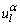is the numerical solution to the radial Schrodinger equation at the energy ε. The coefficients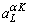are chosen such that the atomic functions for all L components match (in value) the PW with K at the Muffin tin sphere boundary. The KS orbitals are expressed as a linear combinations of APWs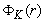. In 1991 Singh  introduced the concept of local orbitals (LOs) which allow an efficient treatment of the semi-core states. An LO is constructed by the LAPW radial functions u andat one energy ε1 in the valence band region and a third radial function at ε2.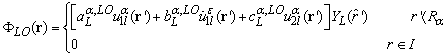(2)

An alternative approach was proposed by Sjöstedt et al , namely the APLW+ lo (local orbital) method. Here the augmentation is similar to the original APW scheme but each radial wavefunction is computed at a fixed linearization energy to avoid the non-linear eigenvalue problem. The missing variational freedom of the radial wavefunctions can be recovered by adding another type of local orbitals (termed in lower case to distinguish them from LO) containing u andterm: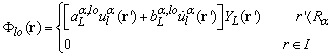(3)

It was demonstrated that this new scheme converges faster than LAPW. The APW +lo scheme has been implemented in the wien2k code version .

However, in the calculations reported here, we chose the muffin tin radii for Si and Sn to be 2.21 a.u and 2.5 a.u. respectively. The expansion of the spherical region is developed up to lmax=10. Furthermore, we have used the energy cut-off of RMTKμαξ=7 and the maximal reciprocal vector equal to 12 for both compounds.

The integrals over the Brillouin zone are performed using the Monkorst-pack special k-points approach . Since calculations of the optical properties require a more dense k-matrix, we have used 1000 k-points in the irreducible Brillouin zone for integration in reciprocal space.

In this paper, three approximations for the exchange-correlation functional have been used, the GGA's of Perdew-Burke-Ernzherhof. (PBE08) , Engel-Vosko (EV93)  and a modified version of the exchange potential proposed by Becke and Johnson (MBJ) is used to calculate the band gaps at different alloy concentrations. The agreement with experiment is very good, this functional was designed to reproduce as well as possible the exact exchange correlation potential rather than the total energy, and as a result gives significantly improved results, this gives us a unique opportunity to test the accuracies of the potentials employed and the calculation schemes in general.

### 3. Results

For the eight atom supercell considered here, there may exist many atomic arrangements for the 50-50% alloy. The unit cell contains four Si atoms and four Sn atoms.

The lattice constant of the alloyAxB1-x (A=Si and B=Sn) are determined using Vegard’s law:Where aA and aB are the lattice constants of the pure semiconductors A and B respectively.

The electronic band structure of the binary alloy SixSn1-x along symmetry lines as a function of mole fraction x is shown in Figure 1 (a ,b, c), Figure 2 (a, b, c) and Figure 3 (a, b, c) for Si, α-Sn and Si0.5Sn0.5 respectively using the three approximations for the exchange-correlation functional mentioned above. The results are shown along with other theoretical values in Table 1. It is found that for the generalized gradient approximation the GGA's of Perdew-Burke-Ernzherhof. (PBE08), the energy gap is underestimated as compared to the experimental value due to the well known artifact of the local density approximation calculations, while the Engel-Vosko scheme gives quite a nice band gap compared to the experimental one, the exchange potential proposed by Becke and Johnson (MBJ) gives significantly improved results.

It is interesting to compare our calculated gaps with experimental data (Table 1). Quasi-particle excitations are not taken into account in density functional theory (DFT), the energy gap calculated from DFT tends to be smaller than the experimental one. There are several reasons for this. One is that the LDA and GGA functionals contain the self-interaction error and do not show a derivative discontinuity, which is important when one wants to compare the Kohn Sham band gap with the experimental band gap.In some cases, the wrong ground state is found, as, e.g., in Ge, where the band gap is around 0.7 eV, whereas the LDA Kohn-Sham gap is slightly negative at ambient pressure . The GGA approximation yields only a small improvement. Quasiparticle calculations essentially overcome the underestimate of the band gap as obtained using the LDA. The GW calculations for GaN for instancepredict band structures in much better agreement with experiment; but they are time consuming, and also they treat the d valence states as part of the pseudopotential core in the case of GaN.

As stated above, Becke and Johnson proposed an exchange potential, which was designed to reproduce the exact exchange potential in atoms. This potential, which does not contain any empirical parameter yields very accurate electronic band structures and gaps for various types of semiconductors and insulators. Also, F. Tran and P. Blaha [28, 29, 30] have proposed an improved version of Becke-Johnson potential, The modified Becke-Johnson exchange potential (MBJ).

Therefore under favorable conditions, with increasingly developed growth techniques, Si-Sn can have attractive prospects in application for fabricating advanced devices.

#### Table 1. Energy gap of SixSn1-x as a function of x, using different methodsDownload asPowerPoint Slide

Veiw figureView current table in a new window

Interesting features of Figure 1 a, b and c are that a direct band gap appears at x =3/8 equals to 0.28eV, underestimated as expected by (GGA08), while Engel-Vosko scheme yieldsa somewhat improved 0.76eV gap, the modified Becke Johnson scheme significantly improves it and gives a gap equal to 1.097eV, the alloy fundamental gap is nonzero for x ≥ 3/8, also the level Γ is lower in energy than the level at L for x=1/2. However, the gap becomes indirect at X for x:>3/8. Potentially useful properties of Si-Sn system may be deduced from this study. There exists a range of concentration where the gap is direct or indirect. A semiconductor with a direct gap between 0.3<x<0.6 is a potential infrared detector or light emitter. For x>3/8 SixSn1-x has an indirect band gap and hence a potential Gunn oscillator. One noticeable feature is that when x<0.1 SixSn1-x becomes metallic. We find that Si-Sn alloy is Si like indirect material for low concentration of Sn and Sn-like direct material for higher concentration.

In order to understand the calculated band structure in terms of the contributing atomic states, the total density of states (DOS) are plotted in Figure 4 a, b and c for Si, Si0.5Sn0.5 and α-Sn respectively.

Figure 4c. Total Density of states for Si0.5Sn0.5 (GGA-08+Engel-Vosko+MBJ).

The total DOS curves give also an ideaabout the dominant orbital character of the groups of bands in the indicated regions of energy for Si and Sn. The elemental Si and Sn materials have valence band densities of states qualitatively similar to the band structures. The electronic configuration of Silicon is: 1s2 2s2p6 3s2p2 and that of grey Tin [Kr] 4d10 5s2 5p2.

It is clear from the calculations that the upper valence band is dominated by contributions from 2p states for Si and 4d states for Sn , and the lower valence band for both compounds is comprised mainly of 2s states.

The density of states of Si, Si0.5Sn0.5 and Sn can be split into two main broad bands. The energy region between -5 eV to 0eV contains the lowest peaks for the 3 compounds, and most prominent bands in the region 1eV to 5eV, note that as we increase the mole fraction this energy range decreases and is shifted to lower energies and intensity transitions peaks appear to increase and reaches ~ 12eV. The minimum of the density of states occurs at Γ at -0.95 eV for Si, -1.05eV for Si0.5Sn0.5 and -0.99eV for Sn. The conduction bands are more difficult to describe than the valence bands because they are more delocalized and more free-electron like. It is known that there is a large difference in the electronegativity of Si and Sn, it is 1.41 for Si and 1.15 for α-Sn in the Phillips scale  and there is also in difference in their atomic valence s and p orbitals energy , (Es=-4.20eV, Ep=1.71eV for Si and Es=-5.67eV, Ep=1.33eV for α-Sn), therefore a large transfer occurs between Si and Sn.

### Conclusion

The electronic properties of Si and α-Snand the binary alloy SixSn1-xhave been investigated using the wien2k package, Full-potential linearized augmented plane wave (FP-LAPW) approach within the density functional theory (DFT) in the local density approximation (LDA) including the generalized gradient approximation (GGA) was used.

Three approximations for the exchange-correlation functional have been used, the GGA's of Perdew-Burke-Ernzherhof. (PBE08)], Engel-Vosko and a modified version of the exchange potential proposed by Becke and Johnson (MBJ) is used to calculate the band gaps at different alloy concentrations.

The use of PBE08 for the exchange-correlation potential allows only minor improvements to the band gap, while the Engel-Vosko scheme permitted us to obtain an energy gap, in good agreement with the experimental measurement and other theoretical calculations. However, the MBJ eigenvalue spectrum gives an excellent description of the band structures and yields significant improvement to the value of the energy gap as compared with the experimental one. The densities of states have been presented and provide additional evidence of the band structures and also has allowed to analyze the contributing atomic states in SixSn1-x.

### Acknowledgment

The authors would like to thank: P. Blaha, K. Schwarz, G. Madsen, D. Kvasnicka and J. Luitz, for providing the code WIEN2k, An Augmented Plane Wave + Local Orbitals Program for Calculating Crystal Properties (Karlheinz Schwarz, Techn. Universität Wien, Austria), 2001. ISBN 3-9501031-1-2

### References

  Jain, C., Willis J.R., Bullogh R.,A review of theoretical and experimental work on the Structure of GexSi1-x strained layers and superlattices, Adv. Phys. (39) 127.1990.In article  Moontragoon, P., Ikonic Z., Harrison P., Band structure calculation of SiGeSn alloy: achieving direct band gap materials, Semicond. Sci. Technol. (22) 742.2007.In article  Volkov B. A., Electronic properties of narrow gap IV–VI semiconductors, Phys.-Usp. (46) 984.2003.In article  Kshrsagar,A. and Kumbhojkar,N, Empirical pseudo-potential studies on electronic structure of semiconducting quantum dots, Bull. Mater. Sci., (31) 3.297-307. June 2008.In article  Friedel, P., Hybertsen, M. S. and Schlüter, M., Local empirical pseudopotential approach to the optical properties of Si/Gesuperlattices, Phys. Rev. B (39), 7974-7977. 1989.In article  Benkabou, K., Aoumeur, F.Z., Abid, H., Amrane, N, Tight binding calculation of electronic properties of ternary alloy ZnS Se1-x, Physica B (337). 147-153. 2003.In article  Ryu, C. S., Oh, G. Y., and Lee, M. H., Electronic properties of a tight-binding and a Kronig-Penney model of the Thue-Morse chain, Phys. Rev. B (48). 132-141. 1993.In article  Kumar, S., Maurya,T. K and Auluck, S.J., Electronic and optical properties of ordered BexZn1-xSe alloys by the FPLAPW method, Phys.: Condens. Matter (20). 075205. 2008.In article  Kumar,S. et al, Band structure and optical properties of hexagonal In-rich In(x)Al(1-x)N alloys, J. Phys.: Condens. Matter (23). 475801. 2011.In article  Engel,E. and Vosko, S.H., Exact exchange-only potentials and the virial relation as microscopic criteria for generalized gradient approximations, Phys.Rev.B(47).13164. 1993.In article  Becke,A. D. and. Johnson, E. R, A simple effective potential for exchange, J. Chem. Phys. (124). 221101. 2006.In article  Kobayashi, N., Zhu, D.H., Katsumata, H., Kakemoto, H., Hasegawa, M., Hayashi, N.,. Makita,Y., Uekusa, S., Tsukamoto, T., Crystallization of SiSn and SiSnC layers in Si by solid phase epitaxy and ion-beam-induced epitaxy, Nucl. Instr. Eth. Phys. Res. (121) 199-202. 1997.In article  Maruyama,T., Akagi, H., Thin Films of Amorphous Silicon-Tin Alloy Prepared by Radio‐Frequency Magnetron Sputtering, J. Electrochem. Soc. 4350. 1997.In article CrossRef  CharrierA. et al, Contrasted electronic properties of Sn-adatom-based (√3×√3)R30° reconstructions on Si(111), Phys. Rev. B. (64). 115407. 2001.In article  Schwarz, K., Blaha P., Madsen, G.K.H., Electronic structure calculations of solids using the WIEN2K package. Comput. Phys. Commun. (147). 71. 2002.In article  Schwarz, K., Blaha,P., Solid state calculations using WIEN2K, Comput. Mater. Sci. (28) 259. 2003.In article  Ceperley, D.M., Alder, B.I., Ground State of the Electron Gas by a Stochastic Method, Phys. Rev. Lett. (45) 566. 1990.In article  Wimmer, E., Krakauer, H., Weinert, M., Freeman, A.J., Full-potential self-consistent linearized-augmented-plane-wave method for calculating the electronic structure of molecules and surfaces: O2 molecule, Phys.Rev.B (24). 864. 1981.In article  Blaha, P., Schwarz K., Sorantin, P., Trikey, S.B., Full-potential, linearized augmented plane wave programs for crystalline systems, Comput.Phys.Commun.(59). 399. 1990.In article  Andersen, O.K., Linear methods in band theory, Phys.Rev.B (12).3060-3083. 1975.In article  Singh, D., Ground-state properties of lanthanum. Treatment of extended-core states, Phys.Rev.B (43).6388-6392. 1991.In article  Sjöstedt, E., Nordström L., Singh, D.J., An alternative way of linearizing the augmented plane-wave method, Solid State Comm. (114)15-20. 2000.In article  Blaha, P., Schwarz K., Madsen G.K.H., Kvanicka, D. Luitz, J., WIEN2k, An Augmented Plane Wave Plus Local Orbitals Program For Calculating Crystal properties, Vienna University of Technology,Austria 2001.In article PubMed  Monkhorst, H.J., Pack, J.D., Special points for Brillouin-zone integrations, Phys.Rev.B13 (1976) 5188.In article CrossRef  Perdew, J.P., Ruzsinszky, A., Csonka, G.I., Vydrov, O.A., Scuseria, G.E., Constantin, L.A., Zhou, X., Burke, K., Restoring the density-gradient expansion for exchange in solids and surfaces. Phys Rev Lett.(13).136406. 2008.In article  Camargo-Martinez, J. A., Baquero, R., Performance of the modified Becke-Johnson potential for semiconductors, Phys. Rev. B (86). 195106. 2012In article  Nakahara, J., Kobayashi, K. and Fujii, A., Edge Absorption Stimulated by Disorder in Mixed Crystals of Thallous Halides, J. Phys. Soc. Jpn. (37) 1319 .1974.In article  Koller, D., Tran, F. and Blaha, P., Merits and limits of the modified Becke-Johnson exchange potential, Phys. Rev. B (83). 195134. 2011.In article  Schwarz, K., BlahaP. and Trickey, S.B., Electronic structure of solids with WIEN2k, Molecular Physics, (108)3147-3166. 2010.In article  Reshak, A. H., Jamal, M., DFT calculation for elastic constants of orthorhombic structure within WIEN2K code: A new package (ortho-elastic), Journal of Alloys and Compounds (543)147-151. 2012.In article  Straub D., Levy L. and Himpsel, F.J., Conduction Band and Surface State Critical Points in Si: An Inverse Photoemission Study, Phys. Rev. Lett. (54). 142. 1985.In article  Cardona M. and Greenway,D.L., Reflectivity of gray tin in the fundamental absorption region, Phys. Rev. (125)1291. 1962.In article  Bouhafs, B., Benkabou, F., Khelifa, B., Dufour,J.P., Energy band structure calculation of GexSn1-x and Six Sn1-x alloys, Infrared Physics and Technol. (36). 967-972. 1995.In article  Phillips, J.C., Bonds and Bands in semiconductors, Academic Press, New York, 1973.In article  Vogl, P., Hjalmarson H.P., Dow, J.D., A semi-empirical tight-binding theory of the electronic structure of semiconductors, J. Phys. Chem. Solids (44).365. 1983.In article